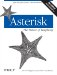# Section 9.4. Writing AGI Scripts in Python

9.4. Writing AGI Scripts in Python

The AGI script we'll be writing in Python , called "The Subtraction Game," was inspired by a Perl program written by Ed Guy and discussed by him at the 2004 AstriCon conference. Ed described his enthusiasm for the power and simplicity of Asterisk when he found he could write a quick Perl script to help his young daughter improve her math skills.

Since we've already written a Perl program using AGI, and Ed has already written the math program in Perl, we figured we'd take a stab at it in Python!

Let's go through our Python script.

` #!/usr/bin/python `

This line tells the system to run this script in the Python interpreter. For small scripts, you may consider adding the - u option to this line, which will run Python in unbuffered mode. This is not recommended, however, for larger or frequently used AGI scripts, as it can affect system performance.

` import sys     import re     import time     import random `

Here, we import several libraries that we'll be using in our AGI script.

` # Read and ignore AGI environment (read until blank line)     env = {}     tests = 0;     while 1:        line = sys.stdin.readline( ).strip( )        if line == '':           break        key,data = line.split(':')        if key[:4] <> 'agi_':           #skip input that doesn't begin with agi_           sys.stderr.write("Did not work!\n");           sys.stderr.flush( )           continue        key = key.strip( )        data = data.strip( )        if key <> '':           env[key] = data     sys.stderr.write("AGI Environment Dump:\n");     sys.stderr.flush( )     for key in env.keys( ):        sys.stderr.write(" -- %s = %s\n" % (key, env[key]))        sys.stderr.flush( ) `

This section of code reads in the variables that are passed to our script from Asterisk, and saves them into a dictionary named env . These values are then written to STDERR for debugging purposes.

` def checkresult (params):        params = params.rstrip( )        if re.search('^200',params):           result = re.search('result=(\d+)',params)           if (not result):              sys.stderr.write("FAIL ('%s')\n" % params)              sys.stderr.flush( )              return -1           else:              result = result.group(1)              #debug("Result:%s Params:%s" % (result, params))              sys.stderr.write("PASS (%s)\n" % result)              sys.stderr.flush( )              return result        else:           sys.stderr.write("FAIL (unexpected result '%s')\n" % params)           sys.stderr.flush( )           return -2 `

The checkresult function is almost identical in purpose to the checkresult subroutine in the sample Perl AGI script we covered earlier in the chapter. It reads in the result of an Asterisk command, parses the answer, and reports whether or not the command was successful.

` def sayit (params):        sys.stderr.write("STREAM FILE %s \"\"\n" % str(params))        sys.stderr.flush( )        sys.stdout.write("STREAM FILE %s \"\"\n" % str(params))        sys.stdout.flush( )        result = sys.stdin.readline( ).strip( )        checkresult(result) `

The sayit function is a simple wrapper around the STREAM FILE command.

` def saynumber (params):        sys.stderr.write("SAY NUMBER %s \"\"\n" % params)        sys.stderr.flush( )        sys.stdout.write("SAY NUMBER %s \"\"\n" % params)        sys.stdout.flush( )        result = sys.stdin.readline( ).strip( )        checkresult(result) `

The saynumber function is a simple wrapper around the SAY NUMBER command.

` def getnumber (prompt, timelimit, digcount):        sys.stderr.write("GET DATA %s %d %d\n" % (prompt, timelimit, digcount))        sys.stderr.flush( )        sys.stdout.write("GET DATA %s %d %d\n" % (prompt, timelimit, digcount))        sys.stdout.flush( )        result = sys.stdin.readline( ).strip( )        result = checkresult(result)        sys.stderr.write("digits are %s\n" % result)        sys.stderr.flush( )        if result:           return result        else:           result = -1 `

The getnumber function calls the GET DATA command to get DTMF input from the caller. It is used in our program to get the caller's answers to the subtraction problems.

` limit=20     digitcount=2     score=0     count=0     ttanswer=5000 `

Here, we initialize a few variables that we'll be using in our program.

` starttime = time.time( )     t = time.time( ) - starttime `

In these lines we set the starttime variable to the current time and initialize t to zero. We'll use the t variable to keep track of the number of seconds that have elapsed since the AGI script was started.

` sayit("subtraction-game-welcome") `

Next , we welcome the caller to the subtraction game.

` while ( t < 180 ):        big = random.randint(0,limit+1)        big += 10        subt= random.randint(0,big)        ans = big - subt        count += 1        #give problem:        sayit("subtraction-game-next");        saynumber(big);        sayit("minus");        saynumber(subt);        res = getnumber("equals",ttanswer,digitcount);        if (int(res) == ans) :           score+=1           sayit("subtraction-game-good");        else :           sayit("subtraction-game-wrong");           saynumber(ans);        t = time.time( ) - starttime `

This is the heart of the AGI script. We loop through this section of code and give subtraction problems to the caller until 180 seconds have elapsed. Near the top of the loop, we calculate two random numbers and their difference. We then present the problem to the caller, and read in the caller's response. If the caller answers incorrectly, we give the correct answer.

` pct = float(score)/float(count)*100;     sys.stderr.write("Percentage correct is %d\n" % pct)     sys.stderr.flush( )     sayit("subtraction-game-timesup")     saynumber(score)     sayit("subtraction-game-right")     saynumber(count)     sayit("subtraction-game-pct")     saynumber(pct) `

After the users are done answering the subtraction problems, they are given their scores.

As you have seen, the basics you should remember when writing AGI scripts in Python are:

• Flush the output buffer after every write. This will ensure that your AGI program won't hang while Asterisk is waiting for the buffer to fill and Python is waiting for the response from Asterisk.

• Write commands to Asterisk with the sys.stdout.write command. Don't forget to call sys.stdout.flush after writing.

#### 9.4.1. The Python AGI Library

If you are planning on writing lot of Python AGI code, you may want to check out Karl Putland's Python module, Pyst . You can find it at http://www. sourceforge .net/projects/pyst/.Asterisk: The Future of Telephony: The Future of Telephony
ISBN: B0026OR3OO
EAN: N/A
Year: 2001
Pages: 380